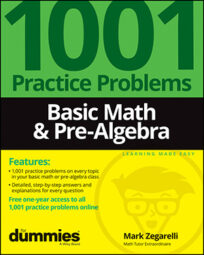##### Basic Math & Pre-AlgebraTo successfully master basic math, you need to practice addition, subtraction, multiplication, and division problems. You also need to understand order of operations, fractions, decimals, percents, ratios, weights and measures, and even a little geometry.

After you've become proficient in these and other basic math concepts, you can begin to tackle pre-algebra, which involves variables, expressions, and equations.

## How to deal with fractions

Fractions are a common way of describing parts of a whole. They’re commonly used for English weights and measures, especially for small measurements in cooking and carpentry. If you want to be proficient in basic math, and if you want to prepare for pre-algebra, you need to know the ins and outs of fractions.

• Remember that the numerator of a fraction is the top number and the denominator is the bottom number.

• The reciprocal of a fraction is that fraction turned upside-down.

• To increase the terms of a fraction, multiply the numerator and denominator by the same number.

• To reduce the terms of a fraction to lowest terms, divide both the numerator and denominator by the greatest possible number.

• To simplify complex fractions, first simplify the numerator and denominator to two separate fractions; then change the problem to division.

## Working with decimals

Decimals are commonly used for money, as well as for weights and measures, especially when using the metric system. As you practice basic math and pre-algebra problems, you’ll find that decimals are easier to work with than fractions.

• To change a decimal to a fraction, put the decimal in the numerator of a fraction with a denominator of 1. Then, continue to multiply both the numerator and denominator by 10 until the numerator is a whole number. If necessary, reduce the fraction.

• To change a fraction to a decimal, divide the numerator by the denominator until the division either terminates or repeats.

• To change a repeating decimal to a fraction, put the repeating portion of the decimal (without the decimal point) into the numerator of a fraction. Use as a denominator a number composed only of 9s with the same number of digits as the numerator. If necessary, reduce the fraction.

• To add or subtract decimals, line up the decimal points.

• To multiply decimals, begin by multiplying without worrying about the decimal points. When you’re done, count the number of digits to the right of the decimal point in each factor and add the result. Place the decimal point in your answer so that your answer has the same number of digits after the decimal point.

• To divide decimals, turn the divisor (the number you’re dividing by) into a whole number by moving the decimal point all the way to the right. At the same time, move the decimal point in the dividend (the number you’re dividing) the same number of places to the right. Then place a decimal point in the quotient (the answer) directly above where the decimal point now appears in the dividend.

• When dividing decimals, continue until the answer either terminates or repeats.

## Figuring percents

Percents are commonly used in business to represent partial amounts of money. They’re also used in statistics to indicate a portion of a data set. As you practice basic math problems, you’ll discover that percents are closely related to decimals, which means that they’re easier to work with than fractions.

• To change a percent to a decimal, move the decimal point two places to the left and drop the percent sign.

• To change a decimal to a percent, move the decimal point two places to the right and attach a percent sign.

• To change a percent to a fraction, drop the percent sign and put the number of the percent in the numerator of a fraction with a denominator of 100. If necessary, reduce the fraction.

• To change a fraction to a percent, first change the fraction to a decimal by dividing. Then change the decimal to a percent by moving the decimal point two places to the right and attaching a percent sign.

• Calculate simple percents by dividing. For example, to find 50% of a number, divide by 2; to find 25%, divide by 4; to find 20%, divide by 5; and so forth.

• You can calculate some percents by reversing the numbers. For example 14% of 50 is the same as 50% of 14, which equals 7.